� Experiment � Calculator/Converters � Radio � Newsletter � Associations and Societies � Component ManufacturersElectronics Symentics A B C D E F G H I J K L M N O P Q R S T U V W X Y Z

Microcontroller Beginner Kit - Learning to use LEDs and Transistors

The LED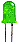An LED is the device shown above. Besides red, they can also be yellow, green and blue. The letters LED stand for Light Emitting Diode. If you are unfamiliar with diodes, take a moment to review the components in the Basic Components Tutorial. The important thing to remember about diodes (including LEDs) is that current can only flow in one direction.

To make an LED work, you need a voltage supply and a resistor. If you try to use an LED without a resistor, you will probably burn out the LED. The LED has very little resistance so large amounts of current will try to flow through it unless you limit the current with a resistor. If you try to use an LED without a power supply, you will be highly disappointed.

So first of all we will make our LED light up by setting up the circuit below.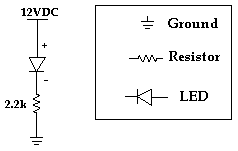Step 1.) First you have to find the positive leg of the LED. The easiest way to do this is to look for the leg that is longer.

Step 2.) Once you know which side is positive, put the LED on your breadboard so the positive leg is in one row and the negative leg is in another row. (In the picture below the rows are vertical.)

Step 3.) Place one leg of a 2.2k ohm resistor (does not matter which leg) in the same row as the negative leg of the LED. Then place the other leg of the resistor in an empty row.

Step 4.) Unplug the power supply adapter from the power supply. Next, put the ground (black wire) end of the power supply adapter in the sideways row with the blue stripe beside it. Then put the positive (red wire) end of the power supply adapter in the sideways row with the red stripe beside it.

Step 5.) Use a short jumper wire (use red since it will be connected to the positive voltage) to go from the positive power row (the one with the red stripe beside it) to the positive leg of the LED (not in the same hole, but in the same row). Use another short jumper wire (use black) to go from the ground row to the resistor (the leg that is not connected to the LED). Refer to the picture below if necessary.

The breadboard should look like the picture shown below.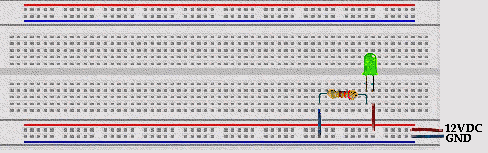Now plug the power supply into the wall and then plug the other end into the power supply adapter and the LED should light up. Current is flowing from the positive leg of the LED through the LED to the negative leg. Try turning the LED around. It should not light up. No current can flow from the negative leg of the LED to the positive leg.

People often think that the resistor must come first in the path from positive to negative, to limit the amount of current flowing through the LED. But, the current is limited by the resistor no matter where the resistor is. Even when you first turn on the power, the current will be limited to a certain amount, and can be found using ohm�s law.

Revisiting Ohm's Law

Ohm's Law can be used with resistors to find the current flowing through a circuit. The law is I = VD/R (where I = current, VD = voltage across resistor, and R = resistance). For the circuit above we can only use Ohm's law for the resistor so we must use the fact that when the LED is on, there is a 1.4 voltage drop across it. This means that if the positive leg is connected to 12 volts, the negative leg will be at 10.6 volts. Now we know the voltage on both sides of the resistor and can use Ohm's law to calculate the current. The current is (10.6 - 0) / 2200 = 0.0048 Amperes = 4.8 mA

This is the current flowing through the path from 12V to GND. This means that 4.8 mA is flowing through the LED and the resistor. If we want to change the current flowing through the LED (changing the brightness) we can change the resistor. A smaller resistor will let more current flow and a larger resistor will let less current flow. Be careful when using smaller resistors because they will get hot.

Next, we want to be able to turn the LED on and off without changing the circuit. To do this we will learn to use another electronic component, the transistor.

1.6.1 The Transistor

Transistors are basic components in all of today's electronics. They are just simple switches that we can use to turn things on and off. Even though they are simple, they are the most important electrical component. For example, transistors are almost the only components used to build a Pentium processor. A single Pentium chip has about 3.5 million transistors. The ones in the Pentium are smaller than the ones we will use but they work the same way.

Transistors that we will use in projects look like this: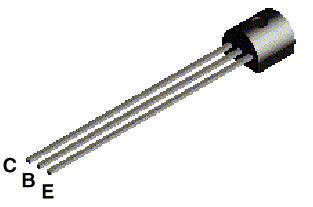The transistor has three legs, the Collector (C), Base (B), and Emitter (E). Sometimes they are labeled on the flat side of the transistor. Transistors always have one round side and one flat side. If the round side is facing you, the Collector leg is on the left, the Base leg is in the middle, and the Emitter leg is on the right.

Transistor Symbol

The following symbol is used in circuit drawings (schematics) to represent a transistor.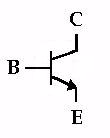Basic Circuit

The Base (B) is the On/Off switch for the transistor. If a current is flowing to the Base, there will be a path from the Collector (C) to the Emitter (E) where current can flow (The Switch is On.) If there is no current flowing to the Base, then no current can flow from the Collector to the Emitter. (The Switch is Off.)

Below is the basic circuit we will use for all of our transistors.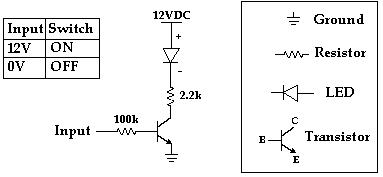To build this circuit we only need to add the transistor and another resistor to the circuit we built above for the LED. Unplug the power supply from the power supply adapter before making any changes on the breadboard. To put the transistor in the breadboard, seperate the legs slightly and place it on the breadboard so each leg is in a different row. The collector leg should be in the same row as the leg of the resistor that is connected to ground (with the black jumper wire). Next move the jumper wire going from ground to the 2.2k ohm resistor to the Emitter of the transistor.

Next place one leg of the 100k ohm resistor in the row with the Base of the transistor and the other leg in an empty row and your breadboard should look like the picture below.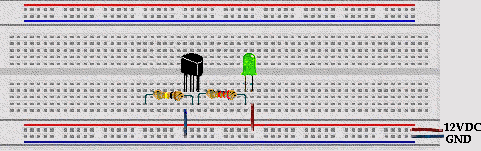Now put one end of a yellow jumper wire in the positive row (beside the red line) and the other end in the row with the leg of the 100k ohm resistor (the end not connected to the Base). Reconnect the power supply and the transistor will come on and the LED will light up. Now move the one end of the yellow jumper wire from the positive row to the ground row (beside the blue line). As soon as you remove the yellow jumper wire from the positive power supply, there is no current flowing to the base. This makes the transistor turn off and current can not flow through the LED. As we will see later, there is very little current flowing through the 100k resistor. This is very important because it means we can control a large current in one part of the circuit (the current flowing through the LED) with only a small current from the input.

Back to Ohm's Law

We want to use Ohm's law to find the current in the path from the Input to the Base of the transistor and the current flowing through the LED. To do this we need to use two basic facts about the transistor.

1.) If the transistor is on, then the Base voltage is 0.6 volts higher than the Emitter voltage.

2.) If the transistor is on, the Collector voltage is 0.2 volts higher than the Emitter voltage.

So when the 100k resistor is connected to 12VDC, the circuit will look like this: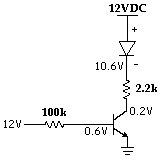So the current flowing through the 100k resistor is (12 - 0.6) / 100000 = 0.000114 A = 0.114 mA.

The current flowing through the 2.2k ohm resistor is (10.6 - 0.2) / 2200 = 0.0047 A = 4.7 mA.

If we want more current flowing through the LED, we can use a smaller resistor (instead of 2200) and we will get more current through the LED without changing the amount of current that comes from the Input line. This means we can control things that use a lot of power (like electric motors) with cheap, low power circuits. Soon you will learn how to use a microcontroller (a simple computer). Even though the microcontroller can not supply enough current to turn lights and motors on and off, the microcontroller can turn transistors on and off and the transistors can control lots of current for lights and motors.

For Ohm�s law, also remember that when the transistor is off, no current flows through the transistor.

1.6.2 Introduction to Digital Devices - The Inverter

In digital devices there are only two values, usually referred to as 0 and 1. 1 means there is a voltage (usually 5 volts) and 0 means the voltage is 0 volts.

An inverter (also called a NOT gate) is a basic digital device found in all modern electronics. So for an inverter, as the name suggests, it's output is the opposite of the input (Output is NOT the Input). If the input is 0 then the output is 1 and if the input is 1 then the output is 0. We can summarize the operation of this device in a table.

 Input Output 1 0 0 1

To help us practice with transistors we will build an inverter. Actually we have already built an inverter. The transistor circuit we just built is an inverter circuit. To help see the inverter working, we will build a circuit with two inverters. The circuit we will use is shown below.

First Inverter (already built)                                                 Second Inverter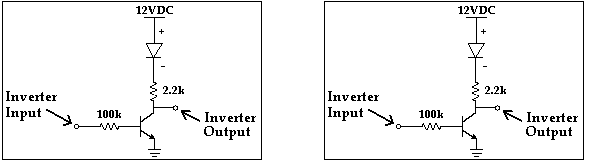To build the circuit, use the transistor circuit we just built as the first inverter. The first inverter input is the end of the 100k ohm resistor connected to the yellow jumper wire. Build another circuit identical to the first (the basic transistor circuit from Section 1.6.1) except leave out the yellow jumper wire connected to the 100k ohm resistor (the inverter input). This circuit is the second inverter.

Connect the output of the first inverter to the input of the second inverter by putting one end of a jumper wire in the same row of holes as the 2.2k ohm resistor and the Collector of the transistor (the output of the first inverter) and putting the other end in the same row of holes as the leg of the 100k ohm resistor of the second inverter (the input to the second inverter).

Here is how to check if you built it correctly. Connect the first inverter input (the yellow jumper wire) to 12V (the positive row). The LED in the first inverter should come on and the LED in the second inverter should stay off. Then connect the first inverter input to 0V (the ground row). (You are turning off the switch of the first inverter.) The first LED should go off and the second LED should come on. If this does not happen, check to make sure no metal parts are touching. Check to make sure all the parts are connected correctly.

The input can either be connected to 12V or 0V. When the Inverter Input is 12V, the transistor in the first inverter will turn on and the LED will come on and the Inverter Output voltage will be 0.2V. The first Inverter Output is connected to the input of the second inverter. The 0.2V at the input of the second inverter is small enough that the second transistor is turned off. The circuit voltages are shown in the diagram below.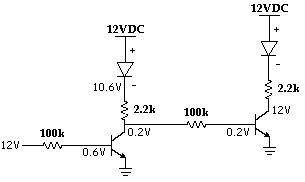When the Inverter Input is connected to 0V, the transistor in the first inverter is turned off and the LED will get very dim. There is a small amount of current still flowing through the LED to the second inverter. The voltage at the first Inverter Output will go up, forcing the second inverter transistor to come on. When the second inverter transistor comes on, the second inverter LED will come on. To find the voltage at the output of the first inverter (10.4V), use Ohm's law. There is no current flowing through the transistor in the first inverter so the path of the current is through the first LED, through the 2.2k resistor, through the 100k resistor, through the second transistor to ground. The voltage at the negative side of the first LED is fixed at 10.6V by the LED. The voltage at the second transistor base is fixed at 0.6V by the transistor. Then given those two voltages, you should be able to find the voltage at the point in the middle (10.4V) using Ohm�s law. (Hint: First find the current and then work through Form 1 of ohm�s law to find the voltage at the point between the 2.2k resistor and the 100k resistor.)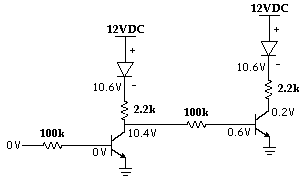Switch the input back and forth from 0V to 12V and you can see that when the first stage is on, the second stage is off. This demonstrates the inverting action of the Inverter.

The next project in the series is called Pulses, Oscillators, Clocks...
It introduces capacitors and the LM555 timer.  With these you can make circuits with LEDs that will continually flash!

Beginner's Kit, includes:

5 - 330 ohm Resistors
5 - 510 ohm Resistors
5 - 2.2K ohm Resistors
5 - 10K ohm Resistors
5 - 100K ohm Resistors
1 - 220 uF Capacitor
5 - LEDs
5 - NPN Transistors
2 - 555 Timer ICs